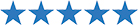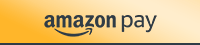# The Fraction Alphabet

\$3.99

### Product RatingN.A.
Not Yet Rated

• Easy, 100% Secure Checkout with## Description

This activity is a unique way for your class to practice fraction operations!

Each student will receive a fraction alphabet key and a worksheet. They will use the key to translate each letter in their name to a fraction. Then, the students will use their “fraction name” to solve fraction operations problems or find the LCD.

## Why did I create the fraction alphabet?

One day while I was working on another fractions resource, I noticed that there were 26 simple fractions between 1/1 and 1/10 if you leave out the sevenths, exactly matching the number of letters in the alphabet.

I ordered the fractions from least to greatest and matched them with the alphabet, creating a “secret code” using fractions.

You can use the fraction alphabet to solve many different types of problems. Pre-select words or have your students select words and then solve by adding, subtracting, multiplying or dividing.

Have a little extra time at the end of class? Use the fraction alphabet to practice fraction operations!

Want to practice with integers? Assign a negative value to all of the vowels!

In addition to the multiple printing options, there’s a conversions chart included so you can use the secret math alphabet to work with decimals and/or percentages instead of only fractions!